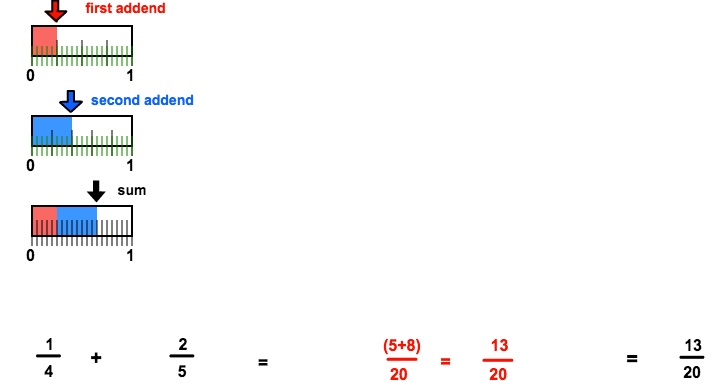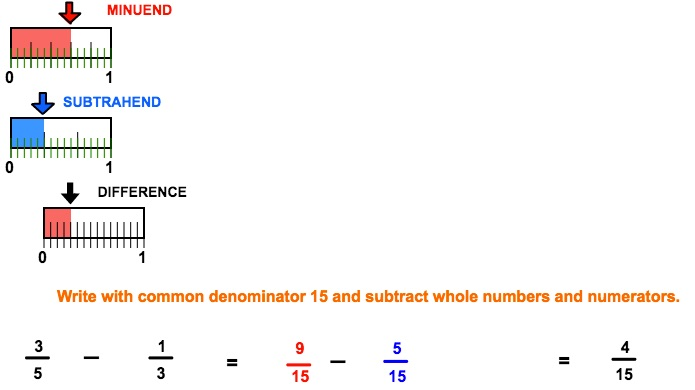# RATL 1 | Lesson 1 | Practice (Solutions: Adding and Subtracting Fractions with Number Lines)

Use multiple number lines to add or subtract:

1. $$\dfrac{1}{4}+\dfrac{2}{5}$$2. $$\dfrac{2}{3}+\dfrac{4}{5}$$3. $$\dfrac{5}{6}-\dfrac{1}{8}$$4. $$\dfrac{3}{5}-\dfrac{1}{3}$$